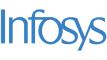New update is available. Click here to update.
Topics

# Palindrome Pairs

Moderate0/80
Average time to solve is 25m+4 more companies

## Problem statement

You are given a list of ‘N’ words ‘WORDS’. Your task is to return all pairs of the distinct indices (i, j) in ‘WORDS’, such that the concatenation of WORDS[i] and WORDS[j] is a palindrome.

For Example:

``````You are given ‘WORDS’ = [“cat”, “mat”, “tac”]. Then the answer will be [(0, 2), (2, 0)}, because “cat” + “tac” = “cattac” which is a palindrome and “tac” + “cat” = “taccat” which is also a palindrome.
``````
Detailed explanation ( Input/output format, Notes, Images )
Constraints:
``````1 <= T <= 10
2 <= N <= 1000
0 <= WORDS[i].LENGTH <= 100
WORDS[i] consists of lowercase English letters

Time Limit: 1 sec
``````
Sample Input 1:
``````2
3
cat
mat
tac
3
ab
ba
bb
``````
Sample Output 1:
``````0 2
2 0
0 1
1 0
``````
Explanation of Sample Input 1:
``````For the first test case, the answer will be [(0, 2), (2, 0)], because “cat” + “tac” = “cattac” which is a palindrome, and “tac” + “cat” = “taccat” which is also a palindrome.

For the second test case, the answer will be [(0, 1), (1, 0)], because “ab” + “ba” = “abba” which is a palindrome, and “ba” + “ab” = “baab” which is also a palindrome.
``````
Sample Input 2:
``````2
2
abc
cba
3
wow
bow
wob
``````
Sample Output 2:
``````0 1
1 0
1 2
2 1
``````Console